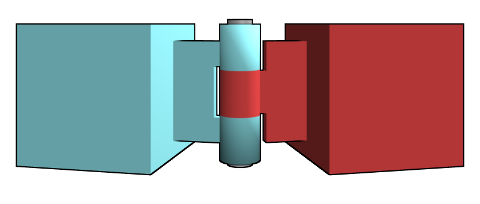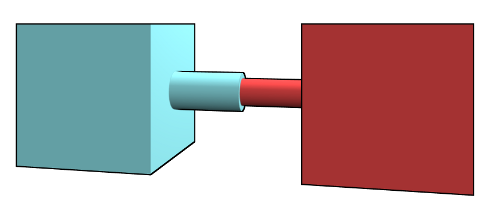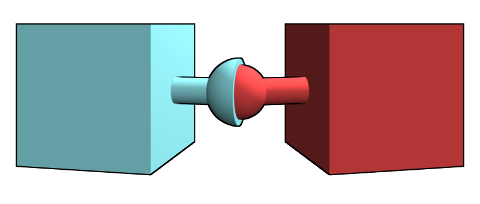# Bullet Constraints

Constraints limit the movement of two rigid bodies in relation to each other, or the movement of one body in relation to the global world space. Another often used term word for constraints is joint.

## Constraint Types

The following different types of constraints are supported by Bullet:

### Hinge Constraint

The hinge constraint has restricts movement of two bodies by means of a shared axis. The axis is defined by a pivot point on each body (within the body’s local space). Hinge constraints can be used for example to model doors or chests.### Slider Constraint

The slider constraint allows the two bodies to move along a shared piston. Rotation around the piston can be limited, if this is required.### Spherical Constraint

The spherical constraint models a ball-and-socket connection between two rigid bodies.### Cone Twist Constraint

The cone twist constraint is a specialized version of the spherical constraint. It allows to limit the rotation and the swing (in both perpendicular directions).

### Generic Constraint

The generic constraint allows movement in all six degrees of freedom, and it allows to limit this movement as desired.

## Constraint between two rigid bodies

All constraints can be created and used in similar ways, so we will explain only one constraint in detail, the `BulletConeTwistConstraint`. For other constraints, please refer to the API documentation.

We assume that we already have created two rigid body nodes, and `npA` and `npB` are NodePaths for these rigid body nodes. For example like the two boxes created in the following snippet

```shape = BulletBoxShape(Vec3(0.5, 0.5, 0.5))

npA = self.worldNP.attachNewNode(BulletRigidBodyNode('A'))
npA.node().setMass(1.0)
npA.setPos(10, 0, 5)
world.attachRigidBody(npA.node())

npB = self.worldNP.attachNewNode(BulletRigidBodyNode('B'))
npB.setPos(10, 0, -5)
self.world.attachRigidBody(npB.node())
```

In the above example body A is dynamic, and body B is static. This means body A will fall down since it is affected by gravity, but body B will always stay where it is. Neither can body B be pushed by dynamic bodies.

Using a cone/twist constraint we can connect body A to body B. The cone/twist constraint will allow body A to move within a cone fixed to body B. Body A will also be able to rotate around the axis from the cone’s vertex point to body A (‘twist’ around this axis).

In order to create the cone/twist constraint we have to define the spatial frames of the cone/twist connector point, as seen from body A and from body B. Then we need to create a new instance of `BulletConeTwistConstraint`, by passing both bodies and both transforms to the constructor. Once created, we can set properties like the scale of the debug visualization of this constraint, as well as limits. Finally, we add the new constraint to the physics world.

```frameA = TransformState.makePosHpr(Point3(0, 0, -5), Vec3(0, 0, -90))
frameB = TransformState.makePosHpr(Point3(0, 0, 5), Vec3(0, 0, -90))

swing1 = 60 # degrees
swing2 = 36 # degrees
twist = 120 # degrees

cs = BulletConeTwistConstraint(npA.node(), npB.node(), frameA, frameB)
cs.setDebugDrawSize(2.0)
cs.setLimit(swing1, swing2, twist)
world.attachConstraint(cs)
```

In this case we have set the following limits:

• Angle of the cone opening in first direction (swing span 1)

• Angle of the cone opening in second direction (swing span 2)

• Maximum twist angle (twist)

In addition we could also add the following parameters: softness, bias factor, relaxation factor.

Which limits are available depends on the constraint type. Please refer to the API documentation.

## Constraint between one rigid body and the world

Adding a constraint between a single body and a fixed point in the global world is similar to adding a constraint between two rigid bodies. The difference is that you pass only one body and one frame to the constructor of the constraint, for example like in the following snippet

```frameA = TransformState.makePosHpr(Point3(0, 0, -5), Vec3(0, 0, -90))

cs = BulletConeTwistConstraint(npA.node(), frameA)
world.attachConstraint(cs)
```